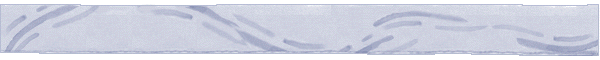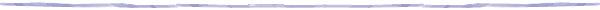# Percolation Theory and Forest Fires

a## Applications of Percolation Theory

Percolation is a model for disordered media. Below, we consider some applications of percolation theory that illustrate the modeling aspects of percolation in diverse situations.

1. Forest fires

The propagation of a fire though a forest can be modeled using percolation. In the simplest model, the forest is represented by a lattice, whose squares are either occupied by a tree or not. Each square can be in one of three states: (a) non burnt tree, (b) a burning tree, or (c) no-tree (either already burnt or there was never a tree there). The spread of fire can be simulated as follows: The ignition point can be set or is chosen randomly to model randomness as is the case of fire ignition by a lightning. Once a tree is burning, there is a probability p that fire will spread to a neighboring tree. As soon as the fire spreads to another tree, the state of that tree changes to "burning" and that tree can now propagate the fire to another neighboring tree. The spread of fire is simulated by marking certain sites in the lattice as burning and following an iterative procedure that checks which other trees or sites are going to burn. Varying the conditions that are used to decide whether a tree propagates the fire to its neighbors (probability p) one can account for static attributes, such as fuel type, elevation and slope, as well as dynamic attributes, such as the direction of the wind or the humidity of the air and the temperature. A cluster in this case is a set of trees corresponding to trees that burnt or are burning. One may be interested in determining the probability that a certain point is reached by the burning cluster. Following this simplistic lattice structure, in the next link  Forest Fire Modeling we explore a more complex structure, the Voronoi structure, for forest representation.

2. Oil fields

Percolation theory can be used as a simple idealized model for predicting the distribution of the oil or gas inside porous rocks or oil reservoirs1. The network considered in this case is formed from the pores filled with oil in a rock. The pores can be connected, which would correspond to open bonds in the percolation model, or can be isolated. To the probability with which a site is occupied in the percolation problem it corresponds the porosity or the average concentration of oil in the rock. In order to obtain a good oil production from a well it is desirable to position it in an area with high porosity. In order to predict the amount of oil that will be produced one needs to estimate the porosity of the rock in the area where the oil reservoir is assumed to be located. To obtain such an estimation, the porosity of rock samples is determined. The difficulty comes from the fact that the samples are usually rock logs with the diameter in the order of centimeters, so in order to obtain the final result one needs to extrapolate the measurement to the scale of the reservoir, which is at least in the order of kilometers. The fundamental question is whether such an extrapolation is legitimate. Percolation theory predicts that this approach is valid when the probability p in the percolation problem is appreciably higher than the percolation threshold pc. On the other hand, if the probability is close to the threshold, even though there might exist an extended cluster that could be quite ramified containing a lot of holes, it is possible that the sample will contain other clusters that unfortunately cannot be reached. In this case the decision to invest would prove to be not profitable.

3. Electrical resistance in a mixture of two media2

Consider mixing two materials A, and B, that are in the form of tiny particles, where A is a conducting material while B is a perfect insulator. Make a compact cube of such a mixture and apply a potential differential to two of the opposite faces of the cube. If the mixture is disordered, it may be reasonable to assume that each tiny particle in the mixture is chosen at random to be of either type A or type B. Neighboring type A particles form clusters that conduct electricity. If such a percolating cluster exists that spans the two opposite faces (electrodes), the electrical resistance of the medium is finite, otherwise is infinite. This in turn depends on the ratio of the particles of type A in the mixture, i.e. probability p. There exists a critical probability, pc, close to .50, such that for p < pc the resistance is infinite, while for p > pc the resistance is finite.

4. Gelation and Polymerization

Consider boiling an egg or more precisely the process through which the yolk and the albumen change their consistency. This phenomenon is in fact gelation, the formation of a network of chemical bonds, which span the whole system. The interest in such problems started with the study of polymerization. The initial attempts tried to understand how small branching molecules form larger and larger macromolecules if more and more chemical molecules are formed between the original molecules. In this situation the initial small molecules correspond to the sites of the lattice and the macromolecules to the clusters of the lattice.1 Stauffer D., Arahony A., 2001, Introduction to Percolation Theory, Taylor & Francis.
2 Grimmet, G., 1999, Percolation, Springer-Verlag.